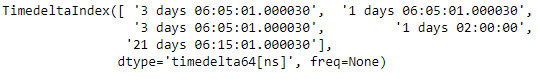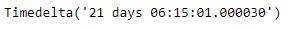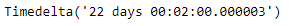# Python | Pandas TimedeltaIndex.max

• Last Updated : 28 Dec, 2018

Python is a great language for doing data analysis, primarily because of the fantastic ecosystem of data-centric python packages. Pandas is one of those packages and makes importing and analyzing data much easier.

Pandas` TimedeltaIndex.max()` function return the maximum value of the TimedeltaIndex object or maximum along an axis.

Attention geek! Strengthen your foundations with the Python Programming Foundation Course and learn the basics.

To begin with, your interview preparations Enhance your Data Structures concepts with the Python DS Course. And to begin with your Machine Learning Journey, join the Machine Learning - Basic Level Course

Syntax : TimedeltaIndex.max(axis=None, *args, **kwargs)

Parameters : None

Return : Timedelta object

Example #1: Use `TimedeltaIndex.max()` function to find the maximum value in the given TimedeltaIndex object.

 `# importing pandas as pd``import` `pandas as pd`` ` `# Create the TimedeltaIndex object``tidx ``=` `pd.TimedeltaIndex(data ``=``[``'3 days 06:05:01.000030'``, ``'1 days 06:05:01.000030'``,``                                ``'3 days 06:05:01.000030'``, ``'1 days 02:00:00'``,``                                                 ``'21 days 06:15:01.000030'``])`` ` `# Print the TimedeltaIndex object``print``(tidx)`

Output :Now we will use the `TimedeltaIndex.max()` function to find the max value in tidx object.

 `# find maximum value``tidx.``max``()`

Output :As we can see in the output, the `TimedeltaIndex.max()` function has returned the maximum value in the given TimedeltaIndex object.

Example #2: Use `TimedeltaIndex.max()` function to find the maximum value in the given TimedeltaIndex object.

 `# importing pandas as pd``import` `pandas as pd`` ` `# Create the TimedeltaIndex object``tidx ``=` `pd.TimedeltaIndex(data ``=``[``'06:05:01.000030'``, ``'3 days 06:05:01.000030'``,``                                ``'22 day 2 min 3us 10ns'``, ``'+23:59:59.999999'``,``                             ``'13 days 06:05:01.000030'``, ``'+12:19:59.999999'``])`` ` `# Print the TimedeltaIndex object``print``(tidx)`

Output :Now we will use the `TimedeltaIndex.max()` function to find the max value in tidx object.

 `# find maximum value``tidx.``max``()`

Output :As we can see in the output, the `TimedeltaIndex.max()` function has returned the maximum value in the given TimedeltaIndex object.

My Personal Notes arrow_drop_up